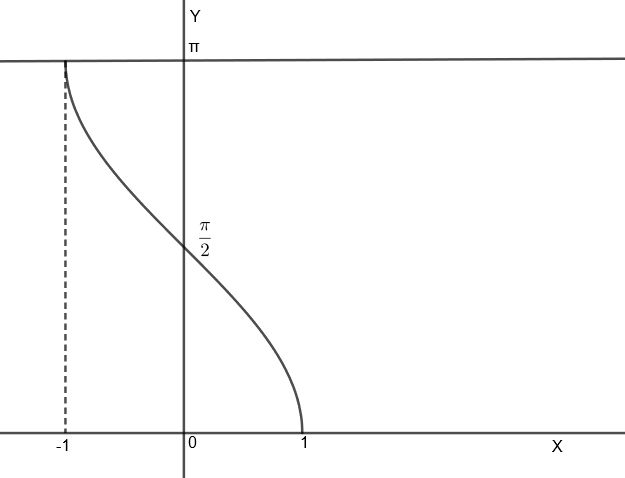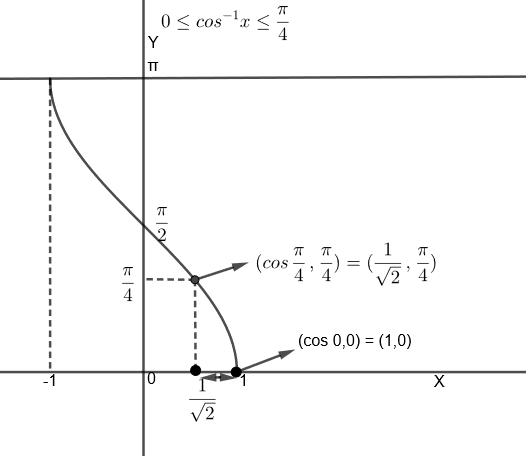Courses
Courses for Kids
Free study material
Free LIVE classes
MoreLIVE
Join Vedantu’s FREE Mastercalss

# $2{{\cos }^{-1}}x={{\sin }^{-1}}\left( 2x\sqrt{1-{{x}^{2}}} \right)$ is valid for all the values of x satisfying(a) $-1\le x\le 1$(b) $0\le x\le 1$(c) $\dfrac{1}{\sqrt{2}}\le x\le 1$(d) $0\le x\le \dfrac{1}{\sqrt{2}}$Verified
362.1k+ views
Hint: We know that the range of ${{\sin }^{-1}}2x\sqrt{1-{{x}^{2}}}$ is $\left[ \dfrac{-\pi }{2},\dfrac{\pi }{2} \right]$, so the same would be for $2{{\cos }^{-1}}x$. From this, find the range of ${{\cos }^{-1}}x$ and then find the value of x for which it is true.

We are given an equation $2{{\cos }^{-1}}x={{\sin }^{-1}}\left( 2x\sqrt{1-{{x}^{2}}} \right)$. We have to solve it and find all the values of x that satisfy this.
Let us consider the equation i.e. : $2{{\cos }^{-1}}x={{\sin }^{-1}}\left( 2x\sqrt{1-{{x}^{2}}} \right)$
We know that the range of ${{\sin }^{-1}}t$ is $\left[ \dfrac{-\pi }{2},\dfrac{\pi }{2} \right]$.
So, we also get the range ${{\sin }^{-1}}\left( 2x\sqrt{1-{{x}^{2}}} \right)$ as $\left[ \dfrac{-\pi }{2},\dfrac{\pi }{2} \right]$
Or, $\dfrac{-\pi }{2}\le {{\sin }^{-1}}\left( 2x\sqrt{1-{{x}^{2}}} \right)\le \dfrac{\pi }{2}$
Since, we know that $2{{\cos }^{-1}}x={{\sin }^{-1}}\left( 2x\sqrt{1-{{x}^{2}}} \right)$ then, $2{{\cos }^{-1}}x$ will also have the same range as ${{\sin }^{-1}}\left( 2x\sqrt{1-{{x}^{2}}} \right)$. So, we get,
$\dfrac{-\pi }{2}\le 2{{\cos }^{-1}}x\le \dfrac{\pi }{2}$
By dividing 2 in the above equation, we get,
$\dfrac{\dfrac{-\pi }{2}}{2}\le \dfrac{2{{\cos }^{-1}}x}{2}\le \dfrac{\dfrac{\pi }{2}}{2}$
Or, $\dfrac{-\pi }{4}\le {{\cos }^{-1}}x\le \dfrac{\pi }{4}$
Now, we will draw the graph of ${{\cos }^{-1}}x$ to analyze the above inequality.Since, we can see that the range of ${{\cos }^{-1}}x$ is $\left[ 0,\pi \right]$, so we will get the range of ${{\cos }^{-1}}x$ in this question as, $0\le {{\cos }^{-1}}x\le \dfrac{\pi }{4}$ because ${{\cos }^{-1}}x$ cannot take negative values.
For ${{\cos }^{-1}}x=0$, we get x = cos 0 = 1.
And for ${{\cos }^{-1}}x=\dfrac{\pi }{4}$, we get $x=\cos \dfrac{\pi }{4}=\dfrac{1}{\sqrt{2}}$.
So, we get, $\dfrac{1}{\sqrt{2}}\le x\le 1$.
Hence, option (c) is the right answer.

Note: In this question, we can also consider the graph of ${{\cos }^{-1}}x$ to solve the inequality
$0\le {{\cos }^{-1}}x\le \dfrac{\pi }{4}$From the above graph, we can see that, when ${{\cos }^{-1}}x\in \left[ 0,\dfrac{\pi }{4} \right]$, then $x\in \left[ \dfrac{1}{\sqrt{2}},1 \right]$. In questions involving the inverse trigonometric functions, take the special case of range and domain of the function.

Last updated date: 29th Sep 2023
Total views: 362.1k
Views today: 5.62k